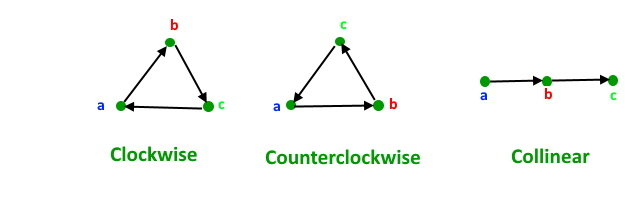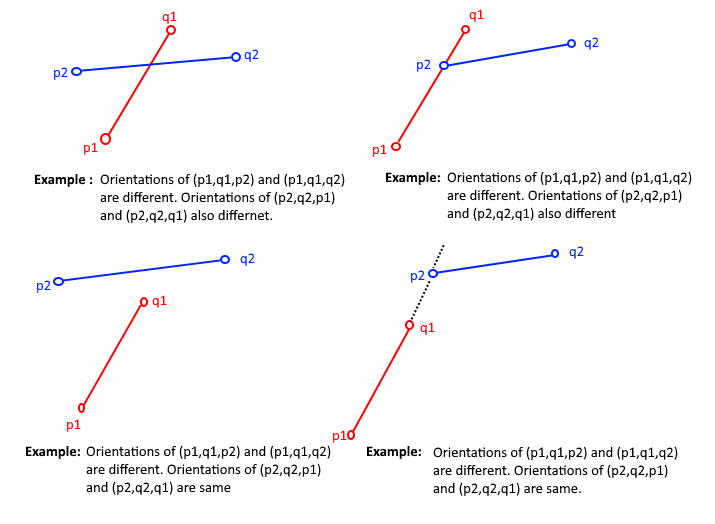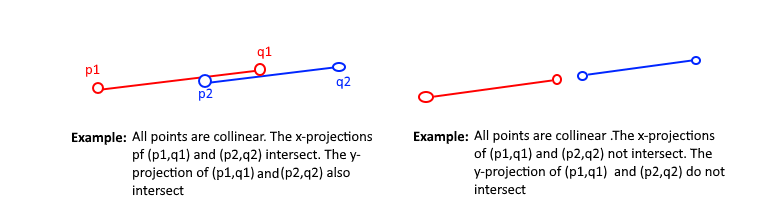# How to check if two given line segments intersect?

Given two line segments (p1, q1) and (p2, q2), find if the given line segments intersect with each other.

Before we discuss solution, let us define notion of orientation. Orientation of an ordered triplet of points in the plane can be
–counterclockwise
–clockwise
–colinear
The following diagram shows different possible orientations of (a, b, c)## Recommended: Please solve it on “PRACTICE” first, before moving on to the solution.

How is Orientation useful here?
Two segments (p1,q1) and (p2,q2) intersect if and only if one of the following two conditions is verified

1. General Case:
– (p1, q1, p2) and (p1, q1, q2) have different orientations and
– (p2, q2p1) and (p2, q2q1) have different orientations.

Examples:2. Special Case
– (p1, q1, p2), (p1, q1, q2), (p2, q2, p1), and (p2, q2, q1) are all collinear and
– the x-projections of (p1, q1) and (p2, q2) intersect
– the y-projections of (p1, q1) and (p2, q2) intersect

Examples:Following is the implementation based on above idea.

## C++

 `// A C++ program to check if two given line segments intersect ` `#include ` `using` `namespace` `std; ` ` `  `struct` `Point ` `{ ` `    ``int` `x; ` `    ``int` `y; ` `}; ` ` `  `// Given three colinear points p, q, r, the function checks if ` `// point q lies on line segment 'pr' ` `bool` `onSegment(Point p, Point q, Point r) ` `{ ` `    ``if` `(q.x <= max(p.x, r.x) && q.x >= min(p.x, r.x) && ` `        ``q.y <= max(p.y, r.y) && q.y >= min(p.y, r.y)) ` `       ``return` `true``; ` ` `  `    ``return` `false``; ` `} ` ` `  `// To find orientation of ordered triplet (p, q, r). ` `// The function returns following values ` `// 0 --> p, q and r are colinear ` `// 1 --> Clockwise ` `// 2 --> Counterclockwise ` `int` `orientation(Point p, Point q, Point r) ` `{ ` `    ``// See https://www.geeksforgeeks.org/orientation-3-ordered-points/ ` `    ``// for details of below formula. ` `    ``int` `val = (q.y - p.y) * (r.x - q.x) - ` `              ``(q.x - p.x) * (r.y - q.y); ` ` `  `    ``if` `(val == 0) ``return` `0;  ``// colinear ` ` `  `    ``return` `(val > 0)? 1: 2; ``// clock or counterclock wise ` `} ` ` `  `// The main function that returns true if line segment 'p1q1' ` `// and 'p2q2' intersect. ` `bool` `doIntersect(Point p1, Point q1, Point p2, Point q2) ` `{ ` `    ``// Find the four orientations needed for general and ` `    ``// special cases ` `    ``int` `o1 = orientation(p1, q1, p2); ` `    ``int` `o2 = orientation(p1, q1, q2); ` `    ``int` `o3 = orientation(p2, q2, p1); ` `    ``int` `o4 = orientation(p2, q2, q1); ` ` `  `    ``// General case ` `    ``if` `(o1 != o2 && o3 != o4) ` `        ``return` `true``; ` ` `  `    ``// Special Cases ` `    ``// p1, q1 and p2 are colinear and p2 lies on segment p1q1 ` `    ``if` `(o1 == 0 && onSegment(p1, p2, q1)) ``return` `true``; ` ` `  `    ``// p1, q1 and q2 are colinear and q2 lies on segment p1q1 ` `    ``if` `(o2 == 0 && onSegment(p1, q2, q1)) ``return` `true``; ` ` `  `    ``// p2, q2 and p1 are colinear and p1 lies on segment p2q2 ` `    ``if` `(o3 == 0 && onSegment(p2, p1, q2)) ``return` `true``; ` ` `  `     ``// p2, q2 and q1 are colinear and q1 lies on segment p2q2 ` `    ``if` `(o4 == 0 && onSegment(p2, q1, q2)) ``return` `true``; ` ` `  `    ``return` `false``; ``// Doesn't fall in any of the above cases ` `} ` ` `  `// Driver program to test above functions ` `int` `main() ` `{ ` `    ``struct` `Point p1 = {1, 1}, q1 = {10, 1}; ` `    ``struct` `Point p2 = {1, 2}, q2 = {10, 2}; ` ` `  `    ``doIntersect(p1, q1, p2, q2)? cout << ``"Yes\n"``: cout << ``"No\n"``; ` ` `  `    ``p1 = {10, 0}, q1 = {0, 10}; ` `    ``p2 = {0, 0}, q2 = {10, 10}; ` `    ``doIntersect(p1, q1, p2, q2)? cout << ``"Yes\n"``: cout << ``"No\n"``; ` ` `  `    ``p1 = {-5, -5}, q1 = {0, 0}; ` `    ``p2 = {1, 1}, q2 = {10, 10}; ` `    ``doIntersect(p1, q1, p2, q2)? cout << ``"Yes\n"``: cout << ``"No\n"``; ` ` `  `    ``return` `0; ` `} `

## Java

 `// Java program to check if two given line segments intersect ` `class` `GFG  ` `{ ` ` `  `static` `class` `Point ` `{ ` `    ``int` `x; ` `    ``int` `y; ` ` `  `        ``public` `Point(``int` `x, ``int` `y)  ` `        ``{ ` `            ``this``.x = x; ` `            ``this``.y = y; ` `        ``} ` `     `  `}; ` ` `  `// Given three colinear points p, q, r, the function checks if ` `// point q lies on line segment 'pr' ` `static` `boolean` `onSegment(Point p, Point q, Point r) ` `{ ` `    ``if` `(q.x <= Math.max(p.x, r.x) && q.x >= Math.min(p.x, r.x) && ` `        ``q.y <= Math.max(p.y, r.y) && q.y >= Math.min(p.y, r.y)) ` `    ``return` `true``; ` ` `  `    ``return` `false``; ` `} ` ` `  `// To find orientation of ordered triplet (p, q, r). ` `// The function returns following values ` `// 0 --> p, q and r are colinear ` `// 1 --> Clockwise ` `// 2 --> Counterclockwise ` `static` `int` `orientation(Point p, Point q, Point r) ` `{ ` `    ``// See https://www.geeksforgeeks.org/orientation-3-ordered-points/ ` `    ``// for details of below formula. ` `    ``int` `val = (q.y - p.y) * (r.x - q.x) - ` `            ``(q.x - p.x) * (r.y - q.y); ` ` `  `    ``if` `(val == ``0``) ``return` `0``; ``// colinear ` ` `  `    ``return` `(val > ``0``)? ``1``: ``2``; ``// clock or counterclock wise ` `} ` ` `  `// The main function that returns true if line segment 'p1q1' ` `// and 'p2q2' intersect. ` `static` `boolean` `doIntersect(Point p1, Point q1, Point p2, Point q2) ` `{ ` `    ``// Find the four orientations needed for general and ` `    ``// special cases ` `    ``int` `o1 = orientation(p1, q1, p2); ` `    ``int` `o2 = orientation(p1, q1, q2); ` `    ``int` `o3 = orientation(p2, q2, p1); ` `    ``int` `o4 = orientation(p2, q2, q1); ` ` `  `    ``// General case ` `    ``if` `(o1 != o2 && o3 != o4) ` `        ``return` `true``; ` ` `  `    ``// Special Cases ` `    ``// p1, q1 and p2 are colinear and p2 lies on segment p1q1 ` `    ``if` `(o1 == ``0` `&& onSegment(p1, p2, q1)) ``return` `true``; ` ` `  `    ``// p1, q1 and q2 are colinear and q2 lies on segment p1q1 ` `    ``if` `(o2 == ``0` `&& onSegment(p1, q2, q1)) ``return` `true``; ` ` `  `    ``// p2, q2 and p1 are colinear and p1 lies on segment p2q2 ` `    ``if` `(o3 == ``0` `&& onSegment(p2, p1, q2)) ``return` `true``; ` ` `  `    ``// p2, q2 and q1 are colinear and q1 lies on segment p2q2 ` `    ``if` `(o4 == ``0` `&& onSegment(p2, q1, q2)) ``return` `true``; ` ` `  `    ``return` `false``; ``// Doesn't fall in any of the above cases ` `} ` ` `  `// Driver code ` `public` `static` `void` `main(String[] args)  ` `{ ` `    ``Point p1 = ``new` `Point(``1``, ``1``); ` `    ``Point q1 = ``new` `Point(``10``, ``1``); ` `    ``Point p2 = ``new` `Point(``1``, ``2``); ` `    ``Point q2 = ``new` `Point(``10``, ``2``); ` ` `  `    ``if``(doIntersect(p1, q1, p2, q2)) ` `        ``System.out.println(``"Yes"``); ` `    ``else` `        ``System.out.println(``"No"``); ` ` `  `    ``p1 = ``new` `Point(``10``, ``1``); q1 = ``new` `Point(``0``, ``10``); ` `    ``p2 = ``new` `Point(``0``, ``0``); q2 = ``new` `Point(``10``, ``10``); ` `    ``if``(doIntersect(p1, q1, p2, q2)) ` `            ``System.out.println(``"Yes"``); ` `    ``else` `        ``System.out.println(``"No"``); ` ` `  `    ``p1 = ``new` `Point(-``5``, -``5``); q1 = ``new` `Point(``0``, ``0``); ` `    ``p2 = ``new` `Point(``1``, ``1``); q2 = ``new` `Point(``10``, ``10``);; ` `    ``if``(doIntersect(p1, q1, p2, q2)) ` `        ``System.out.println(``"Yes"``); ` `    ``else` `        ``System.out.println(``"No"``); ` `} ` `} ` ` `  `// This code is contributed by Princi Singh `

## Python3

 `# A Python3 program to find if 2 given line segments intersect or not ` ` `  `class` `Point: ` `    ``def` `__init__(``self``, x, y): ` `        ``self``.x ``=` `x ` `        ``self``.y ``=` `y ` ` `  `# Given three colinear points p, q, r, the function checks if  ` `# point q lies on line segment 'pr'  ` `def` `onSegment(p, q, r): ` `    ``if` `( (q.x <``=` `max``(p.x, r.x)) ``and` `(q.x >``=` `min``(p.x, r.x)) ``and`  `           ``(q.y <``=` `max``(p.y, r.y)) ``and` `(q.y >``=` `min``(p.y, r.y))): ` `        ``return` `True` `    ``return` `False` ` `  `def` `orientation(p, q, r): ` `    ``# to find the orientation of an ordered triplet (p,q,r) ` `    ``# function returns the following values: ` `    ``# 0 : Colinear points ` `    ``# 1 : Clockwise points ` `    ``# 2 : Counterclockwise ` `     `  `    ``# See https://www.geeksforgeeks.org/orientation-3-ordered-points/amp/  ` `    ``# for details of below formula.  ` `     `  `    ``val ``=` `(``float``(q.y ``-` `p.y) ``*` `(r.x ``-` `q.x)) ``-` `(``float``(q.x ``-` `p.x) ``*` `(r.y ``-` `q.y)) ` `    ``if` `(val > ``0``): ` `         `  `        ``# Clockwise orientation ` `        ``return` `1` `    ``elif` `(val < ``0``): ` `         `  `        ``# Counterclockwise orientation ` `        ``return` `2` `    ``else``: ` `         `  `        ``# Colinear orientation ` `        ``return` `0` ` `  `# The main function that returns true if  ` `# the line segment 'p1q1' and 'p2q2' intersect. ` `def` `doIntersect(p1,q1,p2,q2): ` `     `  `    ``# Find the 4 orientations required for  ` `    ``# the general and special cases ` `    ``o1 ``=` `orientation(p1, q1, p2) ` `    ``o2 ``=` `orientation(p1, q1, q2) ` `    ``o3 ``=` `orientation(p2, q2, p1) ` `    ``o4 ``=` `orientation(p2, q2, q1) ` ` `  `    ``# General case ` `    ``if` `((o1 !``=` `o2) ``and` `(o3 !``=` `o4)): ` `        ``return` `True` ` `  `    ``# Special Cases ` ` `  `    ``# p1 , q1 and p2 are colinear and p2 lies on segment p1q1 ` `    ``if` `((o1 ``=``=` `0``) ``and` `onSegment(p1, p2, q1)): ` `        ``return` `True` ` `  `    ``# p1 , q1 and q2 are colinear and q2 lies on segment p1q1 ` `    ``if` `((o2 ``=``=` `0``) ``and` `onSegment(p1, q2, q1)): ` `        ``return` `True` ` `  `    ``# p2 , q2 and p1 are colinear and p1 lies on segment p2q2 ` `    ``if` `((o3 ``=``=` `0``) ``and` `onSegment(p2, p1, q2)): ` `        ``return` `True` ` `  `    ``# p2 , q2 and q1 are colinear and q1 lies on segment p2q2 ` `    ``if` `((o4 ``=``=` `0``) ``and` `onSegment(p2, q1, q2)): ` `        ``return` `True` ` `  `    ``# If none of the cases ` `    ``return` `False` ` `  `# Driver program to test above functions: ` `p1 ``=` `Point(``1``, ``1``) ` `q1 ``=` `Point(``10``, ``1``) ` `p2 ``=` `Point(``1``, ``2``) ` `q2 ``=` `Point(``10``, ``2``) ` ` `  `if` `doIntersect(p1, q1, p2, q2): ` `    ``print``(``"Yes"``) ` `else``: ` `    ``print``(``"No"``) ` ` `  `p1 ``=` `Point(``10``, ``0``) ` `q1 ``=` `Point(``0``, ``10``) ` `p2 ``=` `Point(``0``, ``0``) ` `q2 ``=` `Point(``10``,``10``) ` ` `  `if` `doIntersect(p1, q1, p2, q2): ` `    ``print``(``"Yes"``) ` `else``: ` `    ``print``(``"No"``) ` ` `  `p1 ``=` `Point(``-``5``,``-``5``) ` `q1 ``=` `Point(``0``, ``0``) ` `p2 ``=` `Point(``1``, ``1``) ` `q2 ``=` `Point(``10``, ``10``) ` ` `  `if` `doIntersect(p1, q1, p2, q2): ` `    ``print``(``"Yes"``) ` `else``: ` `    ``print``(``"No"``) ` `     `  `# This code is contributed by Ansh Riyal `

## C#

 `// C# program to check if two given line segments intersect ` `using` `System; ` `using` `System.Collections.Generic;  ` ` `  `class` `GFG  ` `{ ` ` `  `public` `class` `Point ` `{ ` `    ``public` `int` `x; ` `    ``public` `int` `y; ` ` `  `    ``public` `Point(``int` `x, ``int` `y)  ` `    ``{ ` `        ``this``.x = x; ` `        ``this``.y = y; ` `    ``} ` `     `  `}; ` ` `  `// Given three colinear points p, q, r, the function checks if ` `// point q lies on line segment 'pr' ` `static` `Boolean onSegment(Point p, Point q, Point r) ` `{ ` `    ``if` `(q.x <= Math.Max(p.x, r.x) && q.x >= Math.Min(p.x, r.x) && ` `        ``q.y <= Math.Max(p.y, r.y) && q.y >= Math.Min(p.y, r.y)) ` `    ``return` `true``; ` ` `  `    ``return` `false``; ` `} ` ` `  `// To find orientation of ordered triplet (p, q, r). ` `// The function returns following values ` `// 0 --> p, q and r are colinear ` `// 1 --> Clockwise ` `// 2 --> Counterclockwise ` `static` `int` `orientation(Point p, Point q, Point r) ` `{ ` `    ``// See https://www.geeksforgeeks.org/orientation-3-ordered-points/ ` `    ``// for details of below formula. ` `    ``int` `val = (q.y - p.y) * (r.x - q.x) - ` `            ``(q.x - p.x) * (r.y - q.y); ` ` `  `    ``if` `(val == 0) ``return` `0; ``// colinear ` ` `  `    ``return` `(val > 0)? 1: 2; ``// clock or counterclock wise ` `} ` ` `  `// The main function that returns true if line segment 'p1q1' ` `// and 'p2q2' intersect. ` `static` `Boolean doIntersect(Point p1, Point q1, Point p2, Point q2) ` `{ ` `    ``// Find the four orientations needed for general and ` `    ``// special cases ` `    ``int` `o1 = orientation(p1, q1, p2); ` `    ``int` `o2 = orientation(p1, q1, q2); ` `    ``int` `o3 = orientation(p2, q2, p1); ` `    ``int` `o4 = orientation(p2, q2, q1); ` ` `  `    ``// General case ` `    ``if` `(o1 != o2 && o3 != o4) ` `        ``return` `true``; ` ` `  `    ``// Special Cases ` `    ``// p1, q1 and p2 are colinear and p2 lies on segment p1q1 ` `    ``if` `(o1 == 0 && onSegment(p1, p2, q1)) ``return` `true``; ` ` `  `    ``// p1, q1 and q2 are colinear and q2 lies on segment p1q1 ` `    ``if` `(o2 == 0 && onSegment(p1, q2, q1)) ``return` `true``; ` ` `  `    ``// p2, q2 and p1 are colinear and p1 lies on segment p2q2 ` `    ``if` `(o3 == 0 && onSegment(p2, p1, q2)) ``return` `true``; ` ` `  `    ``// p2, q2 and q1 are colinear and q1 lies on segment p2q2 ` `    ``if` `(o4 == 0 && onSegment(p2, q1, q2)) ``return` `true``; ` ` `  `    ``return` `false``; ``// Doesn't fall in any of the above cases ` `} ` ` `  `// Driver code ` `public` `static` `void` `Main(String[] args)  ` `{ ` `    ``Point p1 = ``new` `Point(1, 1); ` `    ``Point q1 = ``new` `Point(10, 1); ` `    ``Point p2 = ``new` `Point(1, 2); ` `    ``Point q2 = ``new` `Point(10, 2); ` ` `  `    ``if``(doIntersect(p1, q1, p2, q2)) ` `        ``Console.WriteLine(``"Yes"``); ` `    ``else` `        ``Console.WriteLine(``"No"``); ` ` `  `    ``p1 = ``new` `Point(10, 1); q1 = ``new` `Point(0, 10); ` `    ``p2 = ``new` `Point(0, 0); q2 = ``new` `Point(10, 10); ` `    ``if``(doIntersect(p1, q1, p2, q2)) ` `            ``Console.WriteLine(``"Yes"``); ` `    ``else` `        ``Console.WriteLine(``"No"``); ` ` `  `    ``p1 = ``new` `Point(-5, -5); q1 = ``new` `Point(0, 0); ` `    ``p2 = ``new` `Point(1, 1); q2 = ``new` `Point(10, 10);; ` `    ``if``(doIntersect(p1, q1, p2, q2)) ` `        ``Console.WriteLine(``"Yes"``); ` `    ``else` `        ``Console.WriteLine(``"No"``); ` `} ` `} ` ` `  `/* This code contributed by PrinciRaj1992 */`

Output:

```No
Yes
No```